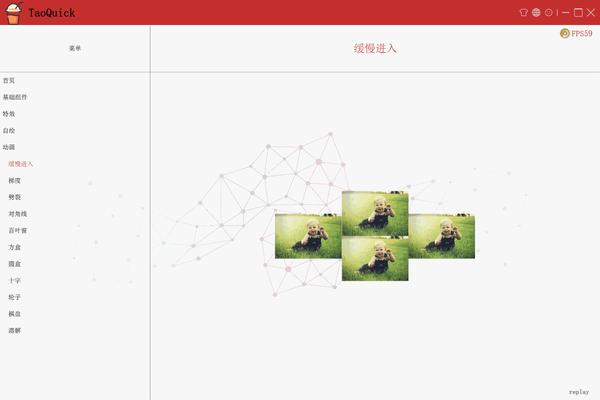• 1631阅读
• 1回复

# Qml特效2-进场动画-梯度 [复制链接]

Qml特效2-进场动画-梯度

(放个目录方便预览。这个目录是从博客复制过来的，点击会跳转到博客)

《Qml组件化编程》系列文章，已经发布了10篇，暂时没有更多内容了，后续可能会更新，看情况。

《Qml特效-页面切换动画》《Qml特效-3D特效》，欢迎大家关注。2. import QtQuick 2.12
3. import QtQuick.Controls 2.12
5.     ...
6.     //枚举声明四种方向
7.     enum Direct {
8.         FromLeft = 0,
9.         FromRight = 1,
10.         FromTop = 2,
11.         FromBottom = 3
12.     }
13.     property int dir: ASlowEnter.Direct.FromLeft
14.     property int percent: 0
15.     opacity: percent > 0 ? 1 : 0
16.     NumberAnimation {
17.         id: animation
18.         target: r
19.         property: "percent"
20.         from: 0
21.         to: 100
22.         alwaysRunToEnd: true
23.         loops: 1
24.         duration: 1000
25.     }
26.     ...
27. }

1. in vec2 qt_TexCoord0;
2. uniform float qt_Opacity;
3. uniform sampler2D effectSource;
4. uniform int dir;
5. uniform int percent;
6. out vec4 fragColor;
7. void main()
8. {
9.     vec4 color = texture2D(effectSource, qt_TexCoord0);
10.     float p = float(percent) / 100.0f;
11.     float alpha = 1.0f;
12.     if (dir == 0 ) {
13.         alpha = 1.0 - step(p, qt_TexCoord0.x);
14.     } else if (dir == 1){
15.         alpha = 1.0 - step(p, 1.0 - qt_TexCoord0.x);
16.     } else if (dir == 2) {
17.         alpha = 1.0f - step(p, qt_TexCoord0.y);
18.     } else if (dir == 3) {
19.         alpha = 1.0f - step(p, 1.0 - qt_TexCoord0.y);
20.     }
21.     fragColor = vec4(color.rgb, alpha);
22. }

step是glsl内置函数，step(p, qt_TexCoord0.x) 就是x小于p返回0，大于等于p返回1。 结果正好与上面分析的相反，用1 减去即可： alpha = 1.0 - step(p, qt_TexCoord0.x);

fragColor = vec4(color.rgb, alpha);

 涛哥是个Qml高手，著有《Qml组件化编程》《Qml特效》系列教程，见知乎专栏-Qt进阶之路：https://zhuanlan.zhihu.com/TaoQt或微信公众号：Qt进阶之路
 分享到 淘江湖 新浪 QQ微博 QQ空间 开心 人人 豆瓣 网易微博 百度 鲜果 白社会 飞信
 1楼 发表于: 2020-04-14限100 字节 您目前还是游客，请 登录 或 注册    回复后跳转到最后一页